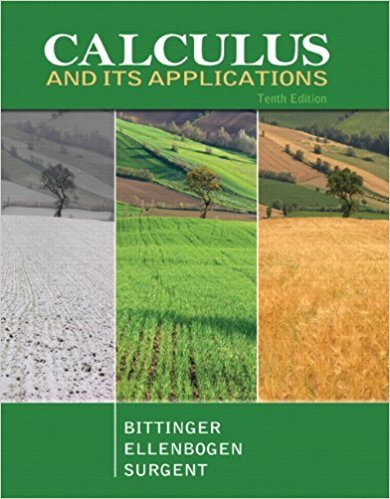×
×

# Solutions for Chapter 4: Integration## Full solutions for Calculus and Its Applications | 10th Edition

ISBN: 9780321694331Solutions for Chapter 4: Integration

Solutions for Chapter 4
4 5 0 417 Reviews
31
1
##### ISBN: 9780321694331

Chapter 4: Integration includes 62 full step-by-step solutions. Since 62 problems in chapter 4: Integration have been answered, more than 24372 students have viewed full step-by-step solutions from this chapter. Calculus and Its Applications was written by and is associated to the ISBN: 9780321694331. This expansive textbook survival guide covers the following chapters and their solutions. This textbook survival guide was created for the textbook: Calculus and Its Applications, edition: 10.

Key Calculus Terms and definitions covered in this textbook
• Angle of elevation

The acute angle formed by the line of sight (upward) and the horizontal

• Boundary

The set of points on the “edge” of a region

• Circular functions

Trigonometric functions when applied to real numbers are circular functions

• Constant term

See Polynomial function

• Cube root

nth root, where n = 3 (see Principal nth root),

• Distributive property

a(b + c) = ab + ac and related properties

• equation of a parabola

(x - h)2 = 4p(y - k) or (y - k)2 = 4p(x - h)

• Graph of a relation

The set of all points in the coordinate plane corresponding to the ordered pairs of the relation.

• Identity function

The function ƒ(x) = x.

• Implicitly defined function

A function that is a subset of a relation defined by an equation in x and y.

• Irreducible quadratic over the reals

A quadratic polynomial with real coefficients that cannot be factored using real coefficients.

• Maximum r-value

The value of |r| at the point on the graph of a polar equation that has the maximum distance from the pole

• Natural numbers

The numbers 1, 2, 3, . . . ,.

• Response variable

A variable that is affected by an explanatory variable.

• Secant line of ƒ

A line joining two points of the graph of ƒ.

• Singular matrix

A square matrix with zero determinant

• Terms of a sequence

The range elements of a sequence.

• Union of two sets A and B

The set of all elements that belong to A or B or both.

• Upper bound for real zeros

A number d is an upper bound for the set of real zeros of ƒ if ƒ(x) ? 0 whenever x > d.

• y-coordinate

The directed distance from the x-axis xz-plane to a point in a plane (space), or the second number in an ordered pair (triple), pp. 12, 629.

×